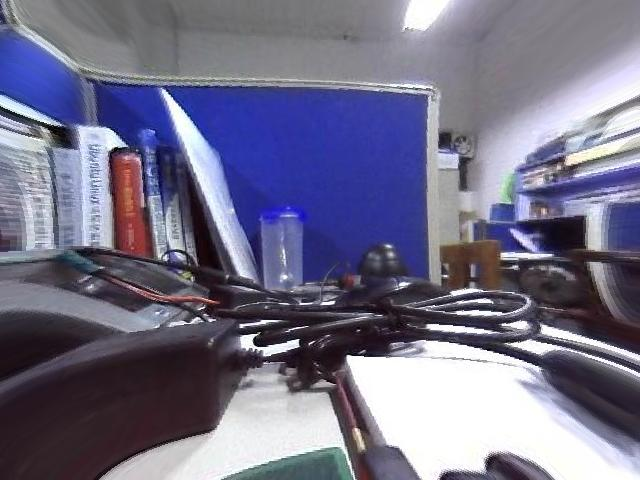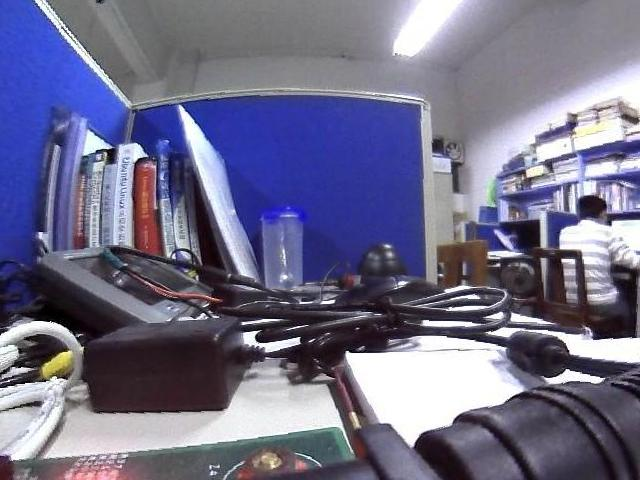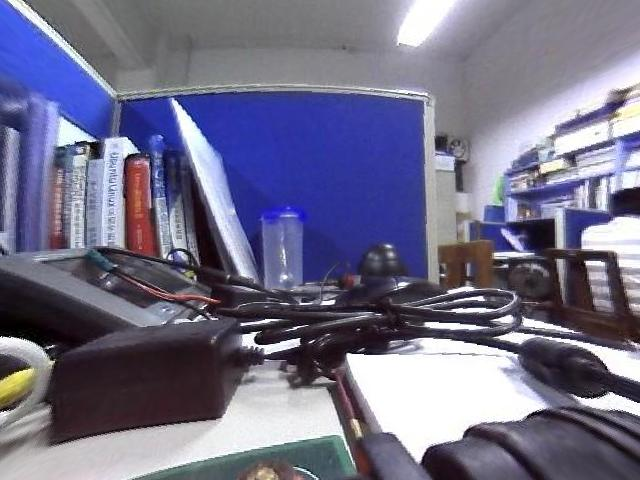# 1        桶形畸变原理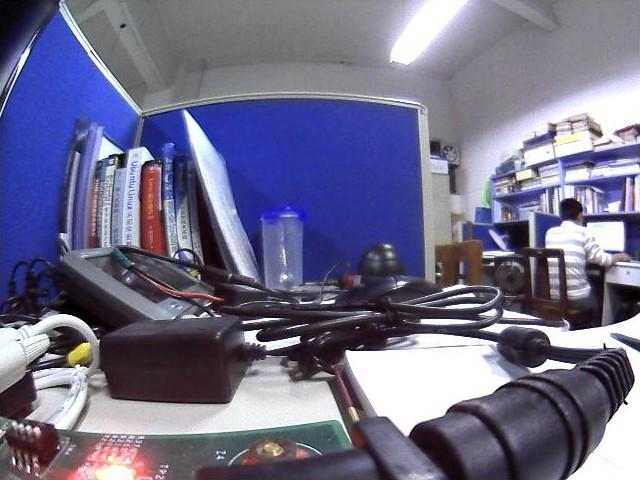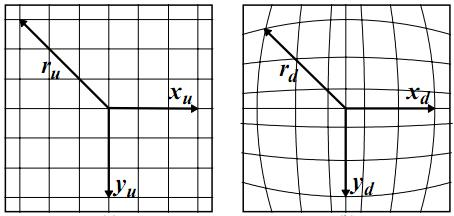2 桶形畸变原理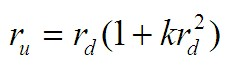（1）

# 2        使用Matlab进行校正

 % Barrel Distortion correction clear; clc;   img_origin = imread('5642_vga.jpg');   % Change this two parameters to improve image quality k1 = -0.0000026; k2 = -0.0000026;   img_size = size( img_origin ); img_undist = zeros( img_size ); img_undist = uint8( img_undist );   for l0 = 1:img_size(3)     for l1 = 1:img_size(1)         y = l1 - img_size(1)/2;         for l2 = 1:img_size(2)             x = l2 - img_size(2)/2;             x1 = round( x * ( 1 + k1 * x * x + k2 * y * y ) );             y1 = round( y * ( 1 + k1 * x * x + k2 * y * y ) );             y1 = y1 + img_size(1)/2;             x1 = x1 + img_size(2)/2;             % if x1 or y1 exceeds boundary， force them to 0(black)             if x1 < 1 || x1 > img_size(2) || y1 < 1 || y1 > img_size(1)                 img_undist(l1,l2,l0) = 0;             else                 img_undist(l1,l2,l0) = img_origin(y1, x1,l0);             end         end     end end   figure(1); % compare the two images in one figure subplot(121); imshow(img_origin); subplot(122); imshow(img_undist);   % save the original image to 1.jpg imwrite(img_origin,'1.jpg'); % save the changed image to 2.jpg imwrite(img_undist,'2.jpg');